# PSEB 5th Class Maths Solutions Chapter 6 Measurement Ex 6.6

Punjab State Board PSEB 5th Class Maths Book Solutions Chapter 6 Measurement Ex 6.6 Textbook Exercise Questions and Answers.

## PSEB Solutions for Class 5 Maths Chapter 6 Measurement Ex 6.6

Question 1.
2 hours 10 min and 1 hour 20 min.
Solution: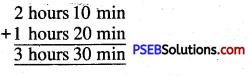Question 2.
4 hours 35 min and 3 hours 40 min.
Solution: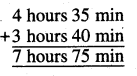= 7 hours + 75 min
= 7 hours + 60 min + 15 min
= 7 hours + 1 hours + 15 min
= 8 hours 15 minQuestion 1.
1 hour 10 min 20 sec and 3 hours 20 min
Solution: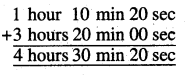Question 2.
2 hours 50 min 30 sec and 1 hour 10 min 30 sec
Solution: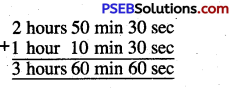3 hours + 60 min + 60 sec
= 3 hours + 1 hour + 1 min + 0 sec
= 4 hours + 1 min + 0 sec
= 4 hours 1 min

Question 1.
7 months and 2 years 3 months
Solution: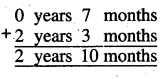Question 2.
4 years 5 months and 1 year 8 months.
Solution: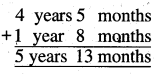= 5 years + 13 months
= 5 years + 12 months + 1 month
= 5 years + 1 year + 1 month
= 6 years 1 month